Courses

# Previous year questions (2016-20) - Laws of Motion Notes | EduRev

## JEE : Previous year questions (2016-20) - Laws of Motion Notes | EduRev

The document Previous year questions (2016-20) - Laws of Motion Notes | EduRev is a part of the JEE Course 35 Years JEE Main & Advanced Question Bank: Physics Class 11.
All you need of JEE at this link: JEE

Q.1. A 60 HP electric motor lifts an elevator having a maximum total load capacity of 2000 kg. If the frictional force on the elevator is 4000 N, the speed of the elevator at full load is close to (1 HP = 746 W, g = 10 m/s2 )    
(a) 1.7 m/s
(b) 1.9 m/s
(c) 1.5 m/s
(d) 2.0 m/s

Given that M = 2000 kg, g = 10 m/s2, f = 4000 N, P = 60 HP = 60 × 746 J
Total force acting on elevator is:
F = Mg + f
= (2000 x 10) + 4000 = 24000 N
Power of electric motor is given by
P = Fv
⇒ v = P/F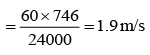Q.2. A mass of 10 kg is suspended by a rope of length 4 m, from the ceiling. A force F is applied horizontally at the mid-point of the rope such that the top half of the rope makes an angle of 45° with the vertical. Then F equals (Take g = 10 m/s2 and the rope to be massless)    
(a) 100 N
(b) 90 N
(c) 70 N
(d) 75 N

Let tension in the string be T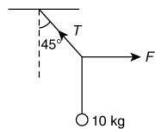At equilibrium, we have
T sin 450 = F     ..(1)
and    T cos450 = 10 x g    ..(2)
Dividing Eq. (1) by Eq. (2), we get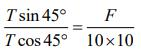(∵ g = 10 m/s)
⇒ I = F/100
⇒ F = 100 N

Q.3. Consider a uniform cubical box of side 'a' on a rough floor that is to be moved by applying minimum possible force F at a point 'b' above its centre of mass (see figure). If the coefficient of friction is μ = 0.4, the maximum possible value of 100.b/a for box not to topple before moving is ______.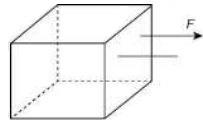Given that μ = 0.4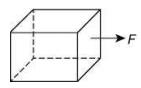Condition for no topple is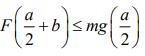Friction force is given by
F = μmg
So,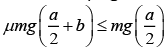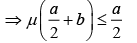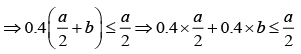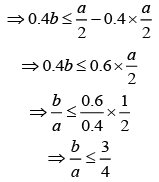So, maximum value of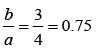Therefore,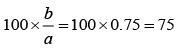So, maximum possible value of 100 × b/a = 75.

Q.4. A spring-mass system (mass m, spring constant k and natural length l) rests in equilibrium on a horizontal disc. The free end of the spring is fixed at the centre of the disc. If the disc together with spring-mass system, rotates about its axis with an angular velocity ω, ( k >> mω2) the relative change in the length of the spring is best given by the option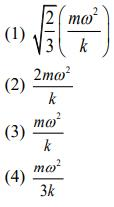Let the elongation in length of the spring be Δl.
Forces act on the system are:
(i) Centripetal force, which act outward which help in expansion in spring:
Fc = mω2 (l + Δl)
(ii) Spring restoring force, which act inward which opposed the further expansion in spring.
Fs = kΔl
At balanced point, we have: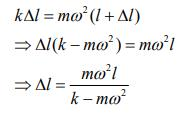The relative change in the length of the spring is: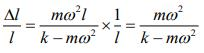Since k>> mωthen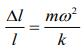Q.5. A block of mass 10 kg is kept on a rough inclined plane as shown in the figure. A force of 3 N is applied on the block. The coefficient of static friction between the plane and the block is 0.6. What should be the minimum value of force P such that the block does not move downward? (Take g = 10 m/s2)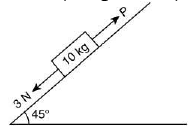(a) 32 N
(b) 18 N
(c) 23 N
(d) 25 N

The coefficient of static friction, μ = 0.6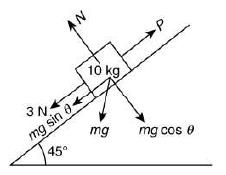The resultant force is
P + μmg cos θ = 3 + mg sin θ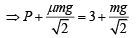Given m = 10 kg, μ = 0.6 and g = 10 m/s2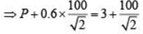⇒ P + 0.6 × 50√2 = 3 + 50√2
⇒ P + 42.42 = 73.71
⇒ P = 73.71 - 42.42 = 31.29
Therefore, P ≈ 32 N

Q.6. A mass of 10 kg is suspended vertically by a rope from the roof. When a horizontal force is applied on the rope at some point, the rope deviated at an angle of 45° at the roof point. If the suspended mass is at equilibrium, the magnitude of the force applied is (g = 10 m/s2).     
(a) 200 N
(b) 140 N

(c) 70 N
(d) 100 N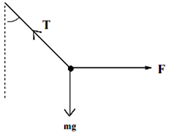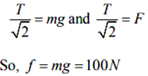Q.7. A particle of mass m is moving in a straight line with momentum p. Starting at time t = 0, a force F = kt acts in the same direction on the moving particle during time interval T so that its momentum changes from p to 3p. Here, k is a constant. The value of T is    
(a)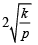(b)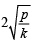(c)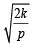(d)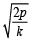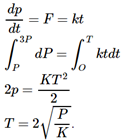Q.8. A block kept on a rough inclined plane, as shown in the figure, remains at rest up to a maximum force 2 N down the inclined plane. The maximum external force up the inclined plane that does not move the block is 10 N. The coefficient of static friction between the block and the plane is (Take g = 10 m/s2)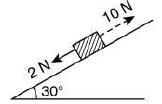(a) √3/2
(b) √3/4
(c) 1/2
(d) 2/3

Block is at rest then, 2 N force is applied.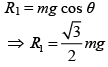Now, friction is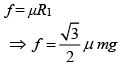When 2 N force is applied in downward direction, thus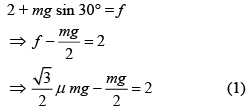Block is rest (10 N force is applied)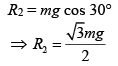When 10 N force is applied in upward direction, then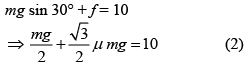Dividing Eq. (1) by Eq. (2), we get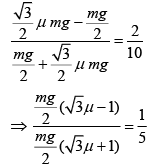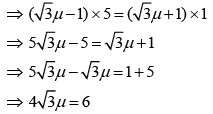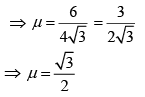Q.9. Two masses m1 = 5 kg and m2 = 10 kg, connected by an inextensible string over a frictionless pulley, are moving as shown in the figure. The coefficient of friction of horizontal surface is 0.15. The minimum weight m that should be put on top of m2 to stop the motion is: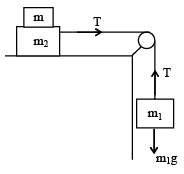(a) 18.3 g

(b) 23.3 kg
(c) 43.3 kg
(d) 10.3 kg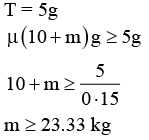Q.10. A given object takes n times more time to slide down a 45° rough inclined plane as it takes to slide down a perfectly smooth 45° incline. The coefficient of kinetic friction between the object and the incline Is: 
(a)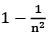(b)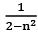(c)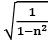(d)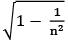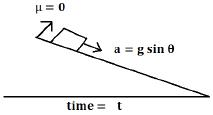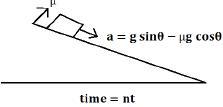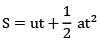So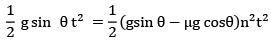Sin θ = n2 sinθ − µn2 cosθ
⇒ 1 = n2 − µn2
⇒ 1 − n2 = −µn2
⇒ n2 − 1 = −µn2
⇒ µ = 1 − 1/n2

Q.11. A man grows into a giant such that his linear dimensions increase by a factor of 9. Assuming that his density remains the same, the stress in the leg will change by a factor of    
(a) 81
(b) 1/81
(c) 9
(d) 1/9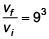∵ Density remains same
So, mass ∝ Volume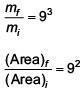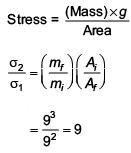Q.12. A body is thrown vertically upwards. Which one of the following graphs correctly represent the velocity vs time?   
(a)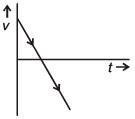(b)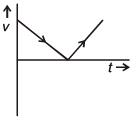(c)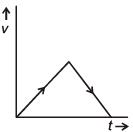(d)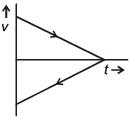Acceleration is constant and negative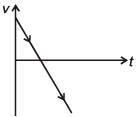Q.13. A uniform disc of radius R and mass M is free to rotate only about its axis. A string is wrapped over its rim and a body of mass m is tied to the free end of the string as shown in the figure. The body is released from rest. Then the acceleration of the body is:(a)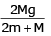(b)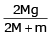(c)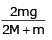(d)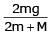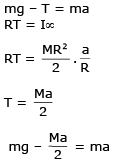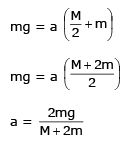Q.14. A conical pendulum of length 1 m makes an angle q = 45° w.r.t. Z-axis and moves in a circle in the XY plane. The radius of the circle is 0.4 m and its center is vertically below O. The speed of the pendulum, in its circular path, will be (Take g = 10 ms-2)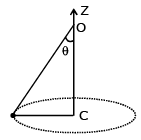(a) 0.2 m/s
(b) 0.4 m/s
(c) 2 m/s
(d) 4 m/s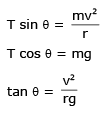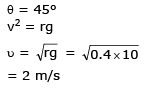Q.15. The machine as shown has 2 rods of length 1 m connected by a pivot at the top. The end of one rod is connected to the floor by a stationary pivot and the end of the other rod has a roller that rolls along the floor in a slot. As the roller goes back and forth, a 2 kg weight moves up and down. If the roller is moving towards right at a constant speed, the weight moves up with a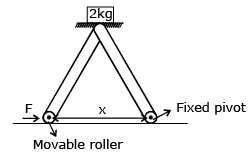(a) Speed which is 3/4th of that of the roller when the weight is 0.4 m above the ground
(b) Constant speed
(c) Decreasing speed
(d) Increasing speed

We know that the velocity is the rate of change in displacement, s with respect to time, t. Here from the diagram we can see that the weight moves up by covering the distance y in time t towards the point C.
Let Vc be the speed (velocity) of the weight moving up towards the point C.
Then, rate of change in y with respect to time is the speed of weight moving up
⇒ dy / dt =  Vc → 1
Vc is the velocity (speed) of the moving up toward point C
Let VA be the velocity (speed) with which the roller moves right, then
⇒ dx / dt =  VA → 2
VA is the velocity (speed) of the moving right
We can understand clearly if we see the diagram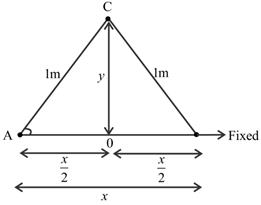From the diagram,
Let us take the triangle AOC, then
⇒ sinθ = opposite side / hypotenuse
⇒ sinθ = y / 1
⇒ sinθ = y
Differentiating above equation,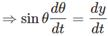From equation 2 we get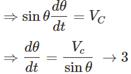Then,
We know that,
⇒ cosθ = adjacent side / hypotenuse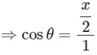⇒ cosθ = x / 2
Differentiating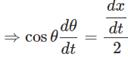From equation 1 we get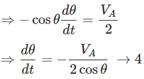From the equation 3 and 4 we get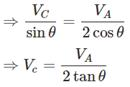It is given that the roller is moving right, if the roller moves rightwards then the angle θ increases, if θ increases then VC will decrease (from the above relation)
Vc is the speed (velocity) of the weight moving up towards the point C.
So, the speed decreases when the weight moves upward.
Hence the correct answer is option (D) decreasing speed
Note : We are saying that if θ increases then VC will decrease from the relation we got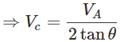We can see that VC is directly proportional to VA and inversely proportional to θ, so if the θ increases then VC will decrease.

Q.16. A point particle of mass m, moves along the uniformly rough track PQR as shown in the figure. The coefficient of friction, between the particle and the rough track equals m. The particle is released, from rest, from the point P and it comes to rest at a point R. The energies, lost by the ball, over the parts, PQ and QR, of the track, are equal to each other, and no energy is lost when particle changes direction from PQ to QR.
The values of the coefficient of friction m and the distance x (= QR), are, respectively close to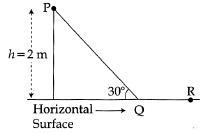(a) 0.2 and 6.5 m
(b) 0.2 and 3.5 m
(c) 0.29 and 3.5 m
(d) 0.29 and 6.5 m

From work energy theorem and given condition: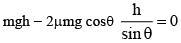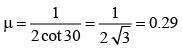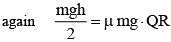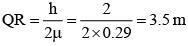Q.17. A rocket is fired vertically from the earth with an acceleration of 2g, where g is the gravitational acceleration. On an inclined plane inside the rocket, making an angle q. With the horizontal, a point object of mass m is kept. The minimum coefficient of friction μmin between the mass and the inclined surface such that the mass does not move is:    
(a) tanθ
(b) tan2θ
(c) 3 tanθ
(d) 2 tanθ

Free body diagram is shown in the figure.
Psuedo force on the object is 2mg in the downward direction as seen from the rocket frame of reference.
Normal force in the direction perpendicular to the inclined plane.
N = 3mg cos(θ)
Maximum frictional force in the direction along the plane,
Fr = μmN cos(θ)
∴ Fr = 3μmmg cos(θ)
This should balance the force on the block along the downward direction of plane.
3mg sin(θ) = 3μmmg cos(θ)
μm = tan(θ)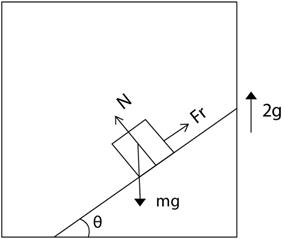Q.18. Which of the following option correctly describes the variation of the speed v and acceleration 'a' of a point mass falling vertically in a viscous medium that applies a force F = –kv, where 'k' is constant, on the body ?( Graphs are schematic and not drawn to scale)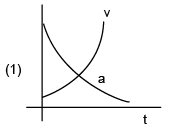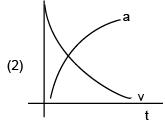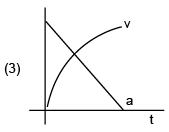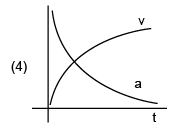a = g – αv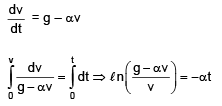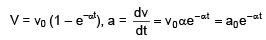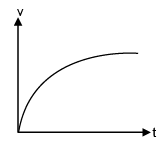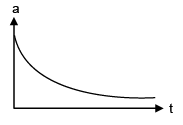Q.19. Concrete mixture is made by mixing cement, stone and sand in a rotating cylindrical drum. If the drum rotates too fast, the ingredients remain stuck to the wall of the drum and proper mixing of ingredients does not take place. The maximum rotational speed of the drum in revolutions per minute (rpm) to ensure proper mixing is close to (Take the radius of the drum to be 1.25m and its axle to be horizontal):    
(a) 1.3
(b) 0.4
(c) 27.0
(d) 8.0

For just complete rotation: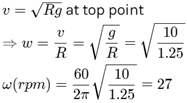Offer running on EduRev: Apply code STAYHOME200 to get INR 200 off on our premium plan EduRev Infinity!

46 docs|30 tests

,

,

,

,

,

,

,

,

,

,

,

,

,

,

,

,

,

,

,

,

,

;